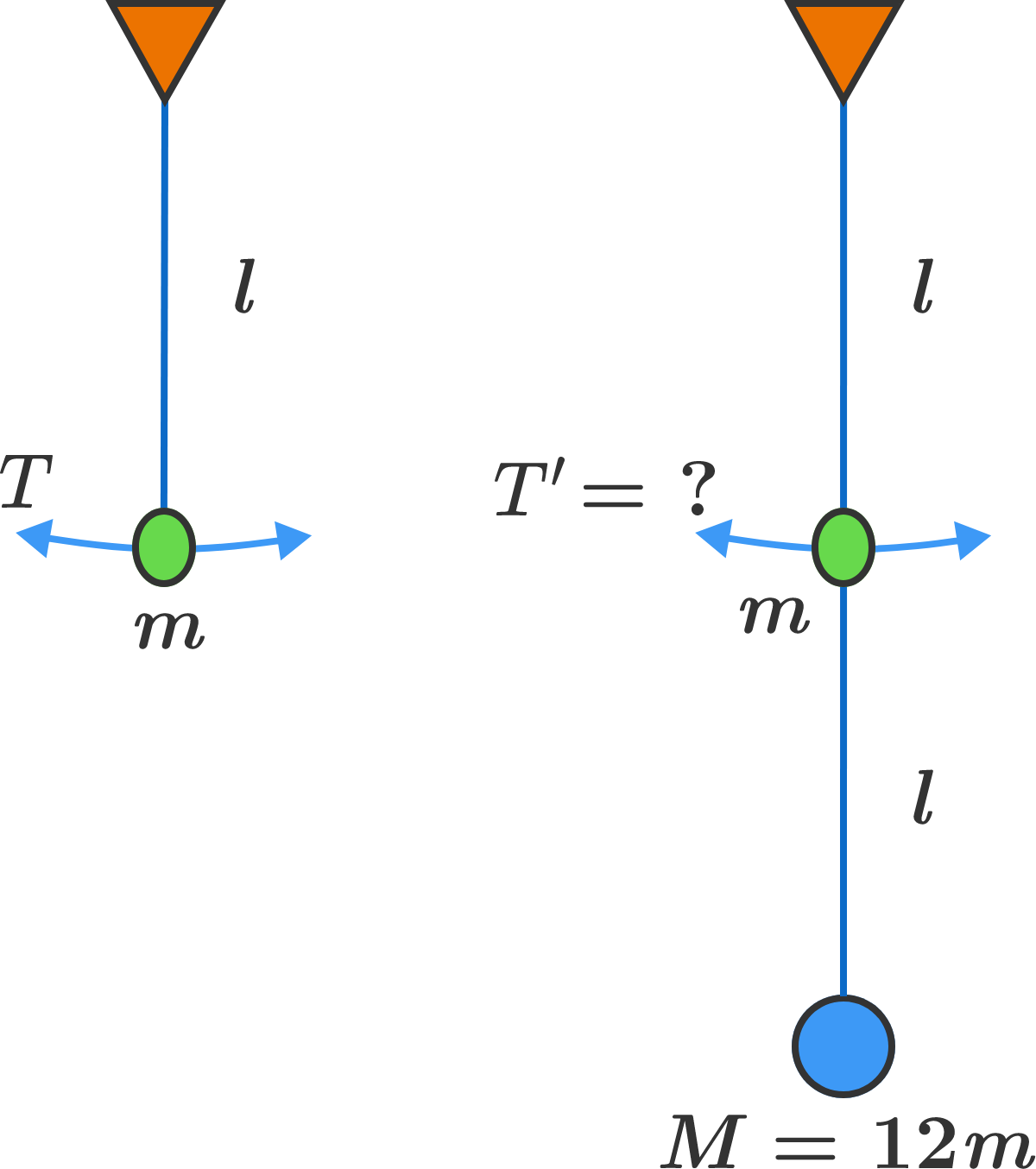# Two-mass pendulum

A simple pendulum of length $\ell$ and bob mass $m$ undergoes small oscillations with the standard period $T = 2\pi\sqrt{\frac{\ell}g}.$

We now attach the second string of length $\ell$, with a bob of mass $12m,$ and hang it from the first bob. If we give a small kick to the bob of mass $m,$ initially the larger mass will hardly move while the small mass will oscillate with period $T^\prime$.

Find $\frac{T^\prime}T.$Note: The ropes are massless and flexible.

×

Problem Loading...

Note Loading...

Set Loading...